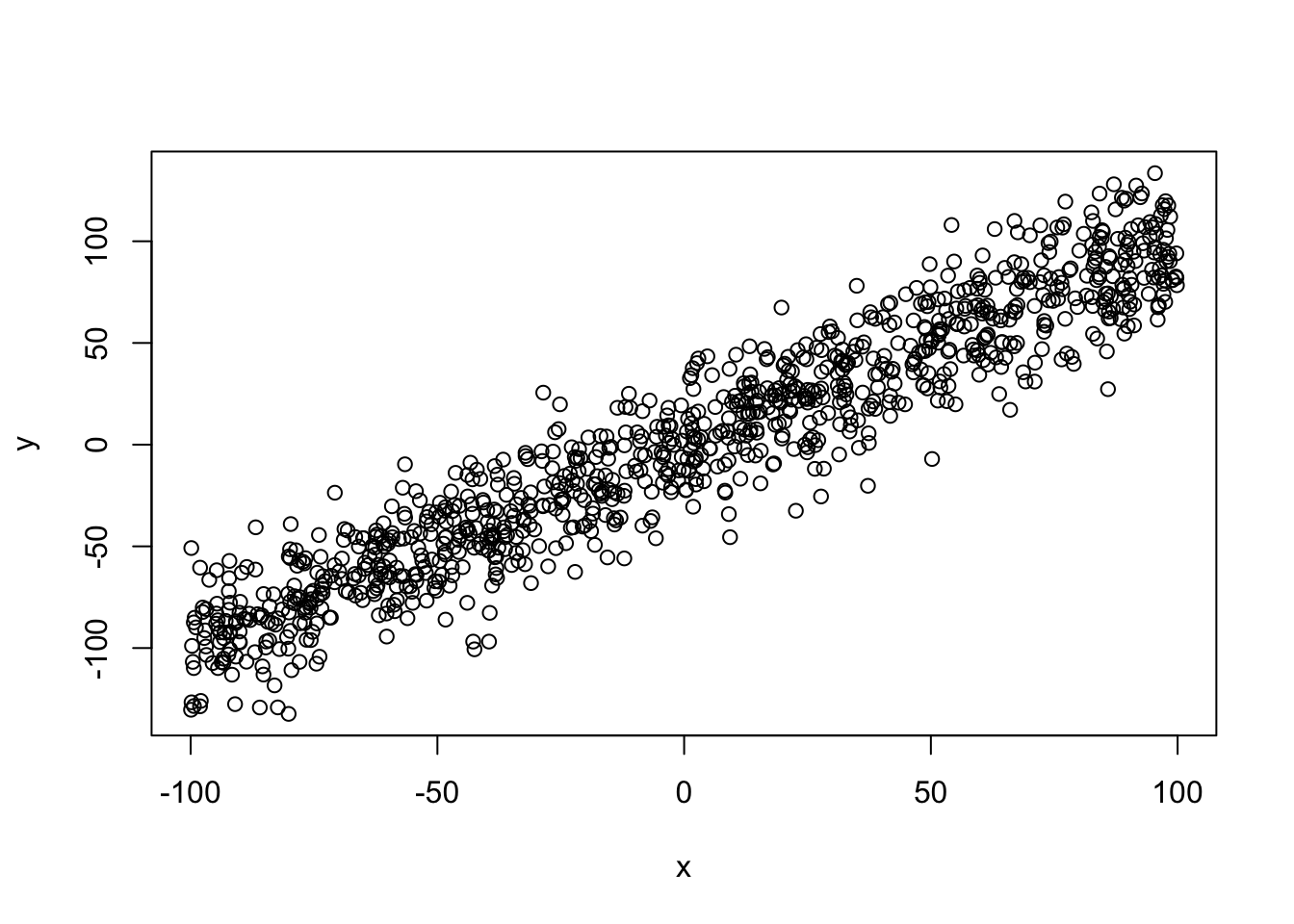pca test

# Question: When transforming vectors to their Principal Components, are their relations preserved?

We have a set of vectors. After performing Principal Component Analysis (PCA) we now use the “rotated” vectors to perform analysis. Can we be confident that the original relations (cosine similarity between vectors) are preserved on the new vector space?

``````knitr::opts_chunk\$set(echo = TRUE)
set.seed(1234)
#Cosine Similarity
cos.sim <- function(A,B)
{
return( sum(A*B)/sqrt(sum(A^2)*sum(B^2)) )
}   ``````

### 1 – Generate a test set y=x+err

``````#"noisy" x=y
x = runif(n = 1000,min=-100,max=100) # x has a uniform distribution
y = x + rnorm(n=1000,mean=0,sd=20) # y has a normal distribution

d <- data.frame(x=x,y=y)
#ver primeros puntos
``````##           x            y
## 1 -77.25932 -57.56371837
## 2  24.45988  -0.03487656
## 3  21.85495  36.04947094
## 4  24.67589  22.49148848
## 5  72.18308 107.83523462
## 6  28.06212  23.19322747``````
``plot(d)``### 2 – Perform PCA

``````pca <- prcomp(d,center = F,scale. = F)
summary(pca)``````
``````## Importance of components:
##                           PC1      PC2
## Standard deviation     83.040 13.30478
## Proportion of Variance  0.975  0.02503
## Cumulative Proportion   0.975  1.00000``````
``pca\$rotation``
``````##        PC1       PC2
## x 0.691987 -0.721910
## y 0.721910  0.691987``````
``````dt <- pca\$x #d transformed
``````##            PC1        PC2
``plot(dt)``